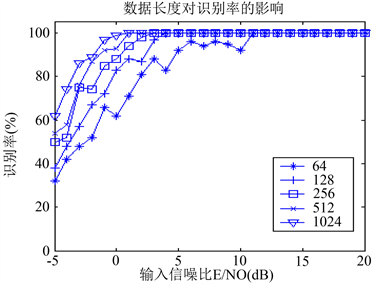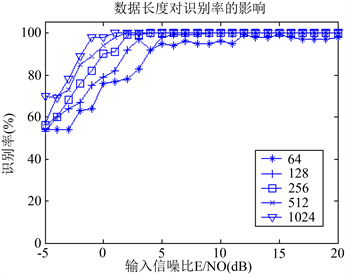﻿ 一种新的MPSK信号识别算法

# 一种新的MPSK信号识别算法A New Algorithm for Classification of MPSK Signals

Abstract: A new algorithm is proposed for classification of MPSK signals. The algorithm is based on wavelet transform and high order cumulants. Compared with other existing algorithms, the proposed al-gorithm reduces the effect of additive Gauass noise and multiplicative noise, therefore, be of more generality. Theoretical analysis and computer simulation justify the robusticity and the efficiency of the new classification algorithm.

1. 引言

2. 数学基础简介

2.1. 信号模型

$x\left(t\right)=s\left(t\right){n}_{m}\left(t\right)\delta \left(t-T\right)+{n}_{e}\left(t\right)=\stackrel{˜}{x}\left(t\right){\text{e}}^{j\left({w}_{c}t+{\theta }_{c}\right)}{n}_{m}\left(t\right)+{n}_{e}\left(t\right)$ (1)

$\stackrel{˜}{x}\left(t\right)=\sqrt{E}\underset{t}{\sum }{e}^{j{\phi }_{i}}{u}_{T}\left(t-iT\right){\phi }_{i}\in {\left\{\frac{2\text{π}}{M}\left(m-1\right)\right\}}_{m=1}^{M}$ (2)

2.2. 高阶累积量的定义及性质

${C}_{40}\left({l}_{1},{l}_{2}\text{},{l}_{3}\right)=\text{cum}\left[x\left(k\right),x\left(k+{l}_{1}\right),x\left(k+{l}_{2}\right),x\left(k+{l}_{3}\right)\right]$ (3)

${C}_{41}\left({l}_{1},{l}_{2}\text{},{l}_{3}\right)=\text{cum}\left[x\left(k\right),x\left(k+{l}_{1}\right),x\left(k+{l}_{2}\right),{x}^{*}\left(k+{l}_{3}\right)\right]$ (4)

${C}_{42}\left({l}_{1},{l}_{2}\text{},{l}_{3}\right)=\text{cum}\left[x\left(k\right),x\left(k+{l}_{1}\right),{x}^{*}\left(k+{l}_{2}\right),{x}^{*}\left(k+{l}_{3}\right)\right]$ (5)

$\text{cum}\left[{a}_{1}{x}_{1},{a}_{2}{x}_{2},\cdots ,{a}_{n}{x}_{n}\right]=\underset{i=1}{\overset{n}{\prod }}{a}_{i}\text{cum}\left[{x}_{1},{x}_{2},\cdots ,{x}_{n}\right]$ (6)

${x}_{1}$${x}_{2}$ 相关，则

$\text{cum}\left[{a}_{1}{x}_{1},{a}_{2}{x}_{2},\cdots ,{a}_{n}{x}_{n}\right]=0$ (7)

${x}_{1}$${y}_{1}$ 相互独立，则

$\text{cum}\left[{x}_{1}+{y}_{1},{x}_{2},\cdots ,{x}_{n}\right]=\text{cum}\left[{x}_{1},{x}_{2},\cdots ,{x}_{n}\right]+\text{cum}\left[{y}_{1},{x}_{2},\cdots ,{x}_{n}\right]$ (8)Table 1. Cumulative amount of MPSK signal

${f}_{{x}_{1}}=\frac{|{C}_{40}\left(0,0,0\right)|}{|{C}_{42}\left(0,0,0\right)|}$${f}_{{x}_{2}}=\frac{|{C}_{41}\left(0,0,0\right)|}{|{C}_{42}\left(0,0,0\right)|}$${F}_{x}=\left[{f}_{x1},{f}_{x2}\right]$ (9)

${F}_{x}$ 就是对MPSK信号星座图的平移、尺度和相位旋转具有不变性的分类特征向量，且对不同阶数的MPSK信号具有如下的形式：

${F}_{\text{PSK},x}=\left\{\begin{array}{cc}\left[1,1\right]& M=2,\text{BPSK}\\ \left[1,0\right]& M=4,\text{QPSK}\\ \left[0,0\right]& M\ge 8,8\text{\hspace{0.17em}}\text{PSK}以上\end{array}$

2.3. 小波变换

$\phi \left(t\right)$ 为尺度函数， $\psi \left(t\right)$ 为母小波，经伸缩平移后得到尺度函数序列 ${\phi }_{j,k}\left(t\right)$ 小波函数序列 ${\psi }_{l,j,k}\left(t\right)$ ，j和k分别为尺度因子和平移因子，对于任意函数 $f\left(t\right)\in {V}_{j}$ 都可以写成如下形式：

$\begin{array}{c}x\left(t\right)=\underset{k=-\infty }{\overset{+\infty }{\sum }}{\phi }_{j,k,l}\left(t\right)+\underset{k=-\infty }{\overset{+\infty }{\sum }}{\psi }_{j,k}\left(t\right)\\ =\underset{k=-\infty }{\overset{+\infty }{\sum }}{c}_{j,k}\left(n\right){\phi }_{j,k}\left(t\right)+\underset{k=-\infty }{\overset{+\infty }{\sum }}{d}_{j,k}\left(n\right){\psi }_{j,k}\left(t\right)\end{array}$ (10)

$\phi \left(t\right)=\sqrt{2}\underset{n}{\sum }h\left(n\right)\phi \left(2t-n\right)$${\psi }_{l}\left(t\right)=\sqrt{2}\underset{n}{\sum }g\left(n\right)\phi \left(2t-n\right)$

${c}_{j+1,k}\left(n\right)=〈x\left(t\right),{\phi }_{j+1,k}\left(t\right)〉=\frac{1}{\sqrt{2}}\underset{n}{\sum }h\left(k-2n\right){c}_{j,k}\left(n\right)$ (11)

${d}_{j+1,k}\left(n\right)=〈x\left(t\right),{\psi }_{j+1,k}\left(t\right)〉=\frac{1}{\sqrt{2}}\underset{n}{\sum }g\left(k-2n\right){c}_{j,k}\left(n\right)$ (12)

2.4. 基于小波的高阶累积量

${W}_{40}\left({l}_{1},{l}_{2}\text{},{l}_{3}\right)=\text{cum}\left[{c}_{j}\left(k\right),{c}_{j}\left(k+{l}_{1}\right),{c}_{j}\left(k+{l}_{2}\right),{c}_{j}\left(k+{l}_{3}\right)\right]$ (13)

${W}_{41}\left({l}_{1},{l}_{2}\text{},{l}_{3}\right)=\text{cum}\left[{c}_{j}\left(k\right),{c}_{j}\left(k+{l}_{1}\right),{c}_{j}\left(k+{l}_{2}\right),{c}_{j}^{*}\left(k+{l}_{3}\right)\right]$ (14)

${W}_{42}\left({l}_{1},{l}_{2}\text{},{l}_{3}\right)=\text{cum}\left[{c}_{j}\left(k\right),{c}_{j}\left(k+{l}_{1}\right),{c}_{j}^{*}\left(k+{l}_{2}\right),{c}_{j}^{*}\left(k+{l}_{3}\right)\right]$ (15)

${W}_{40}\left(0,0,0\right)={\left(\frac{1}{\sqrt{2}}\right)}^{n}{C}_{40}\left(0,0,0\right)\underset{i=0}{\overset{n}{\prod }}{h}_{i}\left(n\right)$ (16)

${W}_{41}\left(0,0,0\right)={\left(\frac{1}{\sqrt{2}}\right)}^{n}{C}_{41}\left(0,0,0\right)\underset{i=0}{\overset{n}{\prod }}{h}_{i}\left(n\right)$ (17)

${W}_{42}\left(0,0,0\right)={\left(\frac{1}{\sqrt{2}}\right)}^{n}{C}_{42}\left(0,0,0\right)\underset{i=0}{\overset{n}{\prod }}{h}_{i}\left(n\right)$ (18)

${F}_{W}=\left[{f}_{{W}_{1}},{f}_{{W}_{2}}\right]=\left[{f}_{{x}_{1}},{f}_{{x}_{2}}\right]$ (19)

${d}_{j,k}=\alpha {d}_{j,k}$$\alpha =\left\{\begin{array}{cc}1& R\le {\chi }_{{\gamma }_{1}}^{2}\\ \beta & R\ge {\chi }_{{\gamma }_{2}}^{2}\\ f\left({\chi }_{\gamma 1}^{2}，{\chi }_{\gamma 2}^{2}\right)& 其他\end{array}$ (20)

1) 对采集信号进行M带小波分解；

2) 对高频和低频部分进行阈值处理；

3) 计算高阶累积量；

4) 利用特征函数进行分类。

3. 仿真结果及讨论Figure 1. The recognition rate for additive Gaussian white noise using method inFigure 2. The recognition rate for additive Gaussian white noise using new algorithmTable 2. The recognition rate for multiplicative noise using method in  (%)Table 3. The recognition rate for multiplicative noise using new algorithm (%)Table 4. The recognition rate for multiplicative and additive mixed noise using method in  (%)Table 5. The recognition rate for multiplicative and additive mixed noise using new algorithm (%)

NOTES

*通讯作者。

 毛用才. 复乘性噪声模型基于累积量的参数估计[J]. 西安电子科技大学学报, 1995, 22(3): 349-354.

 Yang, S.-C. and Chen, W.-D. (2002) Classification of MPSK Signals Using Cumulant Invariants. Journal of Electronics, 19, 99-103.
https://doi.org/10.1007/s11767-002-0018-y

 张贤达. 时间序列分析—高阶统计量方法[M]. 北京: 清华大学出版社, 1996.

 刘振. 基于LabVIEW的信号调制识别研究与应用[D]: [硕士学位论文]. 北京: 北京邮电大学, 2014.

 Leporini, D. and Pesquet, J.-C. (1999) High-Order Wavelet Packets and Cumulant Field Analysis. IEEE Transactions on Information Theory, 45, 863-877.
https://doi.org/10.1109/18.761329

 Ho, K.C., Prokopiw, W. and Chan, Y.T. (2000) Modulation Identification of Digital Signals by the Wavelet Transform. IEE Proceedings-Radar, Sonar and Navigation, 147, 169-176.
https://doi.org/10.1049/ip-rsn:20000492

 陈卫东, 杨绍全, 董春曦, 徐松涛. 多径信道中MPSK信号的调制识别算法[J]. 通信学报, 2002, 23(6): 14-21.

Top